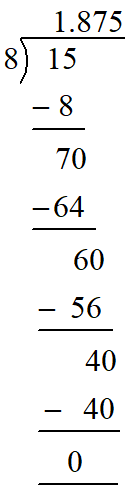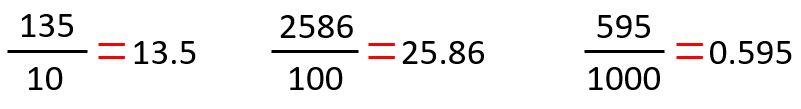Saturday, December 4, 2021
Home > CBSE Class 7 > Fractions

# FractionsHi Students, welcome to Amans Maths Blogs (AMB). In this article, we will study about fractions, which is in the syllabus of Class 7 Maths. It is the notes of fractions chapter which help for the students who study in class 7 in any school.

In this notes, we discuss about definition of fractions, types of fractions, fractions calculator, fractions to decimals, fractions to percentage etc.

So let discuss one by one.

# Fractions

As we have already learnt about the integers and the rational numbers.

The integers are the set of natural numbers, zero and negative of natural number.

For example: 12, -9, 1, 0 etc

And, a number that can be represented in the form of p/q, where p and q are INTEGERS and q ≠ 0 is called as rational numbers. For example: 5/3, -2/5, 16, 2/9 etc.

Now, a fraction can be defined as below.

A number that can be represented in the form of p/q, where p and q are WHOLE numbers and q ≠ 0 is called as fraction. For example: 5/3, 2/5, 1/2, 2/9 etc.

Thus, by the definitions of rational numbers and fractions, we can say that:

RATIONAL NUMBER can be positive or negative, but FRACTION is always positive.

In a fraction p/q (q ≠ 0), the number (p) written over the line known as NUMERATOR and the number (q) written below the line is known as DENOMINATOR is called as fraction.For example: in the fraction 15/22, 15 is the numerator and 22 is the denominator.

# Types of Fractions

There are following types of fractions.## Proper Fractions

A fraction in which the numerator is less than the denominator, then the fraction is known as Proper Fractions.

For example: 3/7, 1/8, 9/11; all are proper fractions.

## Improper Fractions

A fraction in which the numerator is greater than the denominator, then the fraction is known as Improper Fractions.

For example: 12/5, 13/8, 5/2; all are improper fractions.

## Mixed Fractions

A fraction which is addition of a whole number and a proper fraction is known as Mixed Fractions.

For example:; all are mixed fractions.

## Complex Fractions

A fraction which is the fractions of fractions, means the numerator and the denominator, both are fractions is known as Complex Fractions.

For example:is a complex fractions.

A complex fraction (a/b) / (p/q) can be simplified as

(a/b) / (p/q) = (a/b) x (q/p)

Thus,can be simplified as= (1/2) x (5/3) = 5/6

## Equivalent Fractions

A fraction p/q in which the numerator (p) and the denominator (q) is multiplied by a natural number (k) is known as Equivalent Fractions of p/q.

For example:; the fractions 5/7 and 15/21 are equivalent fractions to each other.

## Vulgar Fractions

A fraction p/q in which the denominator (q) is cannot be in the form of 10n, n ∈ N (natural numbers) is known as Vulgar Fractions.

For example: 15/7 is the vulgar fraction as 7 cannot be written in the form of 10n for any natural number n.

## Like Fractions

The fractions with same denominator are known as Like Fractions.

For example: 5/7 and 2/7 are like fractions.

## Unlike Fractions

The fractions with different denominators are known as Unlike Fractions.

For example: 5/7 and 2/3 are unlike fractions.

In this section, we learn how to add two fractions, that may be with same denominator and different denominator.

## Addition of Fractions With Same Denominator

To add two fractions with same denominator, simply we add the numerators and leaves the same denominators.

To understand better the concept of addition of fractions with same denominator, let start with an example:

Suppose we need to addand. In these two fractions, the denominators are same and that is 7.

Thus, to add these two fractions, we simply add the numerators 5 and 13 and we keep the same denominator 7 in the sum.

Therefore, we get## Addition of Fractions With Different Denominator

To add two fractions with different denominators, first we need to find equivalent factions of both the given fractions which have the common denominators.

To get the equivalent fractions with common denominators, we need to find the LCM of the denominators of the given fractions.

And, finally, we add them as the addition of two fractions with same denominators.

To understand better the concept of addition of fractions with different denominators, let start with an example:

Suppose we need to add the fractionsand.

In these two fractions, the denominators are different; 5 and 3.

First we need to find the equivalent fractions ofandwhich have common denominators.

For this, we find the LCM of denominators (5 and 3). LCM (5 and 3) = 15.

Thus, we need to find the equivalent fractions ofandwhich have common denominator as 15.

Now, the equivalent fraction ofwith denominator 15 isand the equivalent fraction ofwith denominator 15 is.

Thus, we get.

To add these fractions, we simply add the numerators 39 and 40.

Thus, we get. Thus, the addition ofandis.

# Fractions Subtractions

In this section, we learn how to subtract two fractions, that may be with same denominator and different denominator.

## Subtraction of Fractions With Same Denominator

To subtract two fractions with same denominator, simply we subtract the numerators and leaves the same denominators.

To understand better the concept of subtraction of fractions with same denominator, let start with an example:

Suppose we need to subtractand. In these two fractions, the denominators are same and that is 7.

Thus, to subtract these two fractions, we simply subtract the numerators 5 and 13 and we keep the same denominator 7 in the difference.

Therefore, we get## Subtraction of Fractions With Different Denominator

To subtract two fractions with different denominators, first we need to find equivalent factions of both the given fractions which have the common denominators.

To understand better the concept of subtraction of fractions with different denominators, let start with an example:

Suppose we need to subtract the fractionsand.

In these two fractions, the denominators are different; 5 and 3.

First we need to find the equivalent fractions ofandwhich have common denominators.

For this, we find the LCM of denominators (5 and 3). LCM (5 and 3) = 15.

Thus, we need to find the equivalent fractions ofandwhich have common denominator as 15.

Now, the equivalent fraction ofwith denominator 15 isand the equivalent fraction ofwith denominator 15 is.

Thus, we get.

# Fractions Multiplication

To multiply the fractions, we simply multiply the numerators of the fractions and the denominators of the fractions whether the fractions have same denominator or different denominator.

And finally, we find the simplest fraction of the multiplication.

(a/b) x (p/q) = (a x p) / (b x q)

To understand better the concept of multiplication of fractions, let start with an example:

Suppose we need to multiply the fractionsand.

Thus,# Fractions Division

To divide the fractions, we multiply one of the fractions to the reciprocal of other fraction.

It means, if a/b and p/q are two fractions, then its division is

(a/b) ÷ (p/q) = (a/b) x (q/p) = (a x q) / (b x p)

To understand better the concept of multiplication of fractions, let start with an example:

Suppose we need to divide the fractionby. Then,# Fractions Simplifications

When more than one mathematical operator are in the expression of fractions, then we need to use PEMDAS rule to simplify the fractions.

Let we understand with an example.

We need to simplify 12/7 x 21 + 15/4 ÷ 3/7 – 4/3.

Thus, by using PEMDAS rule, we get

12/7 x 21 + 15/4 ÷ 3/7 – 4/3

(12/7 x 21) + 15/4 ÷ 3/7 – 4/3 [Multiplication]

= (12 x 3) + 15/4 ÷ 3/7 – 4/3

= 36 + (15/4 ÷ 3/7) – 4/3 [Division]

= 36 + (15/4 x 7/3) – 4/3

= 36 + (5/4 x 7) – 4/3

= 36 + 35/4 – 4/3

= (36 + 35/4) – 4/3 [Addition]

= (179/4 – 4/3) [Subtraction]

= (537 – 16) / 12

= 521 / 12

# Fractions to Decimals

The conversion of a fraction into a decimal is done by the division of a numerator by its denominator. It means, if there is a fraction p/q, then its decimal value can be found by dividing p by q.

Suppose we need to find the decimal value of 15/8. Then, we do it by the long division process of two numbers.Thus, the decimal value of the fraction 15/8 is 1.875.

Take another example of fraction as 25/3 and we need to find its decimal value. For this, again we do the long division process of two numbers as below.Thus, the decimal value of the fraction 25/3 is 8.333… Now, we see the two examples of fraction as 15/8 = 1.875 and 25/3 = 8.333.

Here, we get that the remainder in the division of 15 by 8 is zero while the remainder in the division of 25 by 3 can never be zero.

This is because of 15/8 gives terminating decimal and 25/3 gives non-terminating decimal. It can be found that a fraction gives a terminating or non-terminating decimal without long division.

The fractions with denominator in form of 10n means 10, 100, 1000, 10000 or 10000 etc can be converted into decimal without long division by using following steps:

Step 1: In denominator, count the number of zeros after 1 (in 10, one zero after 1) (in 100, two zero after 2) etc. Let the number of zeroes after 1 is n.

Step 2: In numerator, put the decimal point after n digits from the right.# Fractions to Percentage

The conversion of a fraction (p / q) into percentage is done by multiplying by 100 to p/q and then assign a percentage (%) sign.

p / q = (p/q x 100) %

To understand better the concept of conversion of fractions into percentage, let start with an example:

Suppose we need to convert 3/4 into percentage. For this, we multiply 3/4 and 100 and then simplify it and then assign a % sign.

Thus, we get# Fractions on Number Line

To represent a fraction, let 7/4, we first get the two positive integers in which the given fraction lies, means 7/4 lies between 1 and 2 as 1 < 7/4 < 2.

Now, we mark 1 and 2 on the number line. Since the denominator of 7/4 is 4, then we divide the gap between 1 and 3 into 4 equal parts by marking three lines at equal gap between 1 and 2.

Hence, 1 = 4/4, first mark is 5/4, second mark is 6/4, third mark is 7/4 and 2 = 8/4. We get that the third mark represents 7/4.# Fractions Calculator

If you want to add, subtract, multiply or divide two fractions, you can use the fraction calculator.

Answers are fractions in lowest terms or mixed numbers in reduced form.

To use the fraction calculator, first we input proper or improper fractions and then select the math sign and click Calculate.# Fractions HCF and LCM

The HCF of fractions can be calculated as below.

HCF of Fractions = HCF of Numerators / LCM of Denominators

The LCM of fractions can be calculated as below.

LCM of Fractions = LCM of Numerators / HCF of Denominators

To understand better the concept of conversion of fractions into percentage, let start with an example:

HCF of 3/4 and 9/20 = HCF (3 and 9) / LCM (4 and 20) = 3/20

LCM of 2/9 and 14/45 = LCM (2 and 14) / HCF (9 and 45) = 14/9

AMBiPi
error: Content is protected !!